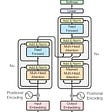## From Deep Learning for Natural Language Processing by Stephan Raaijmakers

Take 40% off Deep Learning for Natural Language Processing by entering fccraaijmakers into the discount code box at checkout at manning.com.

Multitask learning is concerned with learning several things at the same time. An example is to learn both speech tagging and sentiment analysis at the same time, or learning two topic taggers in one go. Why is that a good idea? Ample research has demonstrated, for quite some time already, that multitask learning improves the performance on certain tasks separately. This gives rise to the following application scenario:

You’re training classifiers on a number of NLP tasks, but the performance is disappointing. It turns out your tasks can be decomposed into separate subtasks. Can multitask learning be applied here, and, if it does, does it improve performance on the separate tasks when learned together?

The main motivation for multitask learning is classifier performance improvement. The reason why multitask learning can boost performance is rooted in statistics. Every machine learning algorithm suffers from inductive bias: a set of implicit assumptions underlying its computations. An example of such an inductive bias is the maximization of distances between class boundaries carried out by support vector machines. Another example is the bias in nearest neighbor-based machine learning, where the assumption is that the neighbors (in the feature space) of a specific test data point are in the same class as the test data point. An inductive bias isn’t necessarily a bad thing; it incorporates a form of optimized specialization.

In multitask learning, learning two tasks at the same time -with their own separate inductive biases- produces an overall model that aims for one inductive bias: an inductive bias that optimizes for the two tasks at the same time. This approach may lead to better generalization properties of the separate tasks, meaning that the resulting classifier can handle unseen data better. Often that classifier turns out to be a stronger classifier for both separate tasks.

A good question to ask is which tasks can be combined in such a way that performance on the separate tasks benefits from learning them at the same time. Should these tasks be conceptually related? How to define task relatedness at all? This is a topic beyond the scope of this article. We should focus our experiments on combining tasks that, reasonably, seem to fit together. For instance, named entity recognition may benefit from part of speech tagging, or learning to predict restaurant review sentiment may be beneficial for predicting consumer products sentiment. First, we discuss the preprocessing and handling of our data. After that, we go into the implementation of the three types of multitask learning.

## Data

As mentioned, we use the following datasets for multitask learning:

• Two different datasets for consumer review-based sentiment (restaurant and electronic product reviews).
• The Reuters news dataset, with forty-six topics from the news domain.
• Joint learning of Spanish part of speech tagging and named entity tagging.

For the sentiment datasets, we verify if learning sentiment from two domains in parallel (restaurant and product reviews) improves the sentiment assignment to the separate domains. This is a topic called domain transfer: how to transfer knowledge from one domain to another during learning, in order to supplement small data sets with additional data.

The Reuters news dataset entails a similar type of multitask learning. Given a number of topics, assigned to documents, can we create sensible combinations of pairs of two topics (topics A+B, learned together with topics C+D) that, when learned together, benefit the modeling of the separate topics? And how can we turn such a pairwise discrimination scheme into a multiclass classifier. Below, we find out.

Finally, the last task addresses multitask learning applied to shared data with different labelings. In this task, we create two classifiers, one focusing on part of speech tagging, and the other on named entity recognition. Do these tasks benefit from each other? Let’s take a look at each of our datasets in turn.

## Consumer reviews: Yelp and Amazon

We use two sentiment datasets: sets of Yelp restaurant reviews and Amazon consumer reviews, labeled for positive or negative sentiment. These datasets can be obtained from Kaggle.

The Yelp dataset contains restaurant reviews, with data like:

`The potatoes were like rubber and you could tell they had been made up ahead of time being kept under a warmer.,0   The fries were great too.,1   Not tasty and the texture was just nasty.,0   Stopped by during the late May bank holiday off Rick Steve recommendation and loved it.,1   The Amazon dataset contains reviews of consumer products:   o there is no way for me to plug it in here in the US unless I go by a converter.,0   Good case, Excellent value.,1   Great for the jawbone.,1   Tied to charger for conversations lasting more than 45 minutes.MAJOR PROBLEMS!!,0   The mic is great.,1   I have to jiggle the plug to get it to line up right to get decent volume.,0`

## Data handling

First, let us discuss how to load the sentiment data into our model. The overall schema is the following.

The following procedure converts our data into feature vectors, labeled with integer-valued class labels.

`def loadData(train, test):   global Lexicon   with io.open(train,encoding = "ISO-8859-1") as f:       trainD = f.readlines() ❶   f.close()   with io.open(test,encoding = "ISO-8859-1") as f:       testD = f.readlines() ❷   f.close()   all_text=[]   for line in trainD:       m=re.match("^(.+),[^\s]+\$",line)       if m:         all_text.extend(m.group(1).split(" ")) ❸     for line in testD:       m=re.match("^(.+),[^\s]+\$",line)       if m:         all_text.extend(m.group(1).split(" ")) ❹   Lexicon=set(all_text) ❺   x_train=[]   y_train=[]   x_test=[]   y_test=[]   for line in trainD: ❻       m=re.match("^(.+),([^\s]+)\$",line)       if m:         x_train.append(vectorizeString(m.group(1),Lexicon))         y_train.append(processLabel(m.group(2)))   for line in testD: ❼       m=re.match("^(.+),([^\s]+)\$",line)       if m:         x_test.append(vectorizeString(m.group(1),Lexicon))         y_test.append(processLabel(m.group(2)))   return (np.array(x_train),np.array(y_train)),(np.array(x_test),np.array(y_test)) ❽`

❶ Read the training data into an array of lines.

Similar for the test data.

Extend the all_text array with training data. We need this for a lexicon for vectorization of our data.

Similar for the test data.

Build a lexicon.

Vectorize the training data (see below for vectorizeString), using the lexicon.

Similar for test data.

Return the vectorized training and test data.

The vectorizeString function converts a string into a vector of word indices, using a lexicon. It’s based on the familiar one_hot function of Keras we have encountered before:

Listing 2: Vectorizing strings.

`def vectorizeString(s,lexicon):     vocabSize = len(lexicon)     result = one_hot(s,round(vocabSize*1.5))     return result`

The processLabel function creates a global dictionary for the class labels in the dataset:

Listing 3: Creating a class label dictionary.

`def processLabel(x):     if x in ClassLexicon:         return ClassLexicon[x]     else:         ClassLexicon[x]=len(ClassLexicon)         return ClassLexicon[x]`

The final processing of the data takes place after this: padding the feature vectors to uniform length, and converting the integer-based class labels to binary vectors with the Keras built-in to_categorical:

y_train = keras.utils.to_categorical(y_train, num_classes) y_test = keras.utils.to_categorical(y_test, num_classes)

Now that our data is in place, let us establish a baseline result: what does a standard, single-task classifier produce for these datasets? Here’s our single-task setup:

`(x_train,y_train),(x_test,y_test)=loadData(train,test) ❶ num_classes=len(ClassLexicon) ❶   epochs = 100 batch_size=128 max_words=len(Lexicon)+1   max_length = 1000 x_train = pad_sequences(x_train, maxlen=max_length, padding='post') ❷ x_test = pad_sequences(x_test, maxlen=max_length, padding='post')   y_train = keras.utils.to_categorical(y_train, num_classes) ❸ y_test = keras.utils.to_categorical(y_test, num_classes)   inputs=Input(shape=(max_length,)) ❹ x=Embedding(300000, 16)(inputs) ❺ x=Dense(64,activation='relu')(x) ❻ x=Flatten()(x) ❼ y=Dense(num_classes,activation='softmax')(x) ❽   model=Model(inputs=inputs, outputs=y) ❾ model.compile(loss='categorical_crossentropy',               optimizer='adam',               metrics=['accuracy'])   history = model.fit(x_train, y_train,                     batch_size=batch_size,                     epochs=epochs,                     verbose=1,                     validation_split=0.1)`

Pad training and test data to a pre-specified length.

Convert the labels to a one-hot vector (binary, categorical) representation.

Our input layer.

Input data is embedded with a 300,000 words embedding, producing 16-dimensional vectors.

Create a dense layer with output dimension of 64.

Flatten the data, and add a dense layer.

Pass the dense layer output to a softmax output layer, producing class probabilities.

Create the model, and fit it on the data.

Running this model on Amazon and Yelp produces the following accuracy scores:

• Amazon: 77.9%
• Yelp: 71.5%

These single-task scores are our baseline. Does multitask learning improve on these scores?

If you want to find out, you’ll have to check out the book on Manning’s liveBook platform here.

--

--

--

## More from Manning Publications

Love podcasts or audiobooks? Learn on the go with our new app.

## How to learn Machine Learning in 2022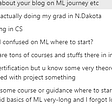## Potential Applications of Semi-Supervised and Self-Supervised Learning for Automated Map Making and…## TensorFlow for Machine Learning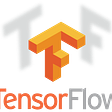## Now Convert you free text to python code : Poor Man’s AI Programmer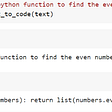## How to use AI Machine Learning in B2B Marketplace## How to Create Products for Your Machine Learning Startup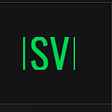## Human Pose Estimation with Deep Neural Networks## Great Ways To Implement MLOPs## A BERT Flavor to Sample and Savor## NLP — From Word Embedding to Transformers## N-gram Language Models | Part 1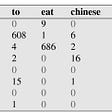## Language Models, Pre-training and Transformers